# 基于caffe与MATLAB接口回归分析与可视化

2017/01/26 20:56

-----------------更新日志-----------------

20:33 2017/1/28    提供MATLAB生成loss曲线的方法

-----------------更新日志-----------------

# 通过caffe进行回归分析

## 通过MATLAB准备HDF5数据

MATLAB是正经的MATLAB，比如你的数据含有35个输入变量，共10000个 样本；先在MATLAB中创建一个变量，将数据（在此之间别忘了先做scaling）拷贝进去，将得到一个10000x 35的变量，当然标签变量就是10000 x 1的啦；最终得到train_x（10000 x 35）、train_y（10000 x 1）、test_x（600 x 35）、test_y（600 x 1）共四个变量，将他们保存到mat文件中，比如data.mat文件。

%% WRITING TO HDF5
filename           =   'train.h5';
num_total_samples  =   size(train_x,1);            %获取样本总数
data_disk          =   train_x';
label_disk         =   train_y';
chunksz            =   100;                        %数据块大小
created_flag       =   false;
totalct            =   0;
for batchno=1:num_total_samples/chunksz
fprintf('batch no. %d\n', batchno);
% 在dump到hdf5文件之前模拟内存中放置的最大数据量
% 存入hdf5
startloc        =   struct('dat',[1,totalct+1], 'lab',[1,totalct+1]);
curr_dat_sz     =   store2hdf5(filename, batchdata,batchlabs, ~created_flag, startloc, chunksz);
created_flag    =   true;                       %设置flag为了只创建一次文件
totalct         =   curr_dat_sz(end);           %更新数据集大小 (以样本数度量)
end
%显示所存储HDF5文件的结构体
h5disp(filename);
%创建HDF5_DATA_LAYER中使用的list文件
FILE               =   fopen('train.txt', 'w');
fprintf(FILE, '%s', filename);
fclose(FILE);
fprintf('HDF5 filename listed in %s \n','train.txt');


## 网络设计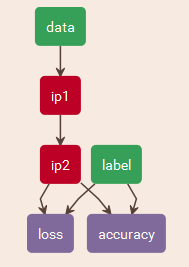## 训练和测试

1、添加头文件#include<direct.h>

_mkdir("./log/");
FLAGS_colorlogtostderr =true;                               //设置输出到屏幕的日志显示相应颜色
//如下为设置各类日志信息对应日志文件的文件名前缀
FLAGS_max_log_size = 1024;                                  //最大日志大小为 1024MB
FLAGS_stop_logging_if_full_disk =true;                      //磁盘写满时，停止日志输出


（1）将日志复制到CAFFE_ROOT\tools\extra目录下（可以看到同目录下的py程序），接着在该目录下打开命令行，并运行命令：

md logconv
set logfile=log_info_20170116-163216.9556
python parse_log.py %logfile% ./logconv
ren logconv\%logfile%.train %logfile%.csv
pause（2）通过如下代码进行提取，可以保存成parse_log.m文件，正则表达式语法和Python的基本相同，稍微改动了一下，功能较caffe提供的有所阉割，毕竟有的暂时用不到。

function []= parse_log(filename)
regex_iteration_str = 'Iteration (\d+)';
regex_train_output_str = 'Train net output #(\d+): (\S+) = ([.\deE+-]+)';
FILE	=   fopen(filename);
FOUT	=   fopen(sprintf('%s.csv',filename),'w');
fprintf(FOUT,'iter,accuracy,loss\n');
plotdata=[];
while ~feof(FILE)
aline   =   fgetl(FILE);
rescell     =   regexpi(aline,regex_iteration_str,'tokens');
if size(rescell,1)~=0;
iteration   =   int32(str2num(rescell{1,1}{1,1}));
end
rescell     =   regexpi(aline,regex_train_output_str,'tokens');
if size(rescell,1)~=0;
if str2num(rescell{1,1}{1,1})==0
accuracy    =   str2num(rescell{1,1}{1,3});
end
if str2num(rescell{1,1}{1,1})==1
loss    =   str2num(rescell{1,1}{1,3});
plotdata(size(plotdata,1)+1,1)   =	iteration;
plotdata(size(plotdata,1),2:3)   =	[accuracy loss];
fprintf(FOUT,'%d,%f,%f\n',iteration,accuracy,loss);
end
end
end
fclose(FILE);
fclose(FOUT);
figure,plot(plotdata(:,1),plotdata(:,2),'LineSmoothing','on');
xlabel('iter'),ylabel('accuracy');
figure,plot(plotdata(:,1),plotdata(:,3),'LineSmoothing','on');
xlabel('iter'),ylabel('loss');
end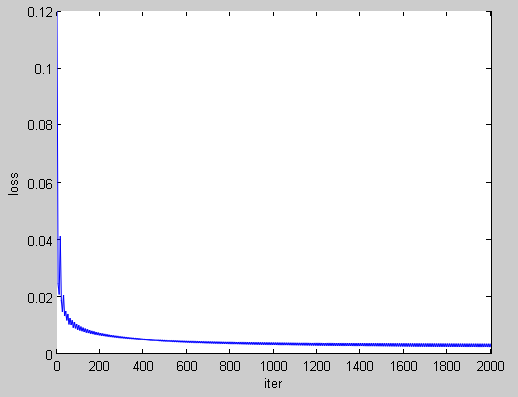# 基于MATLAB的模型可视化

## deploy文件编写

1、*_deploy.prototxt文件的构造没有test网络中的test模块，只有训练模块

include{  phase：TRAIN/TEST  }

2、数据层的写法更加简洁：

input: "data"
input_dim: 1
input_dim: 3
input_dim: 32
input_dim: 32

3、卷积层和全连接层中weight_filler{}与bias_filler{}两个参数不用再填写，因为这两个参数的值由已经训练好的模型caffemodel文件提供。

4、输出层的变化没有了test模块测试精度，具体输出层区别如下：

*_train_test.prototxt文件：
layer{
name: "loss"
type: "SoftmaxWithLoss" #注意此处与下面的不同
bottom: "ip2"
bottom: "label" #注意标签项在下面没有了，因为下面的预测属于哪个标签，因此不能提供标签
top: "loss"
}
*_deploy.prototxt文件：
layer {
name: "prob"
type: "Softmax"
bottom: "ip2"
top: "prob"
}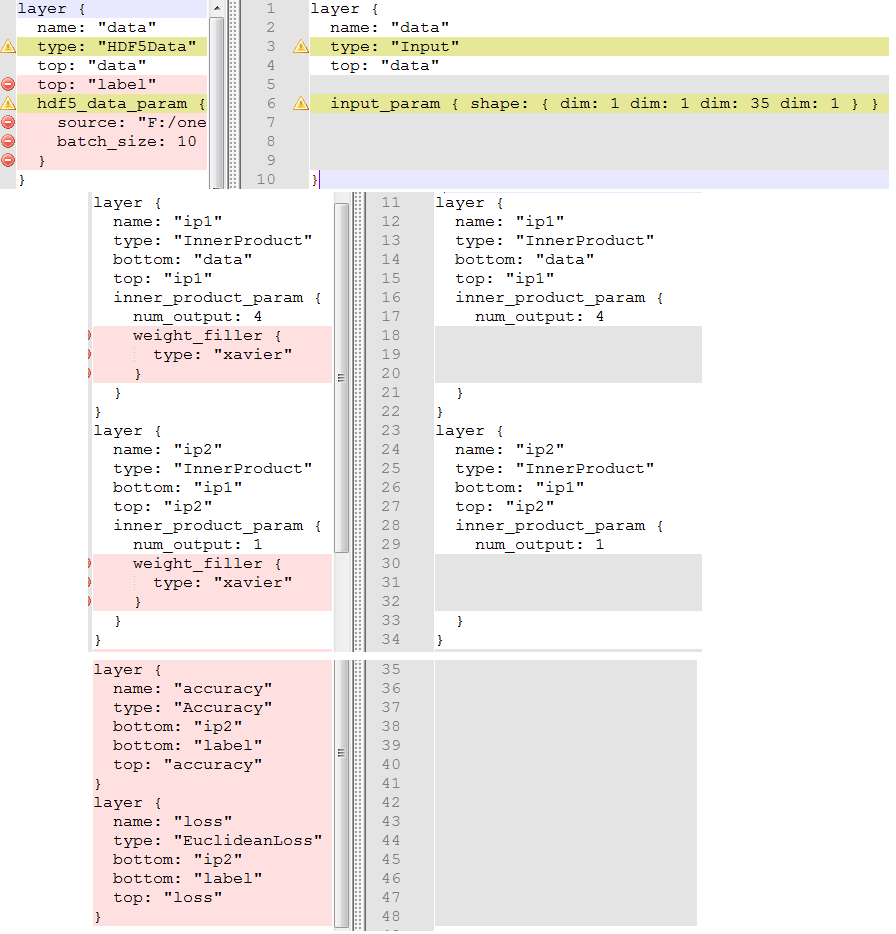## 通过MATLAB接口读取训练好的模型文件

load 'data.mat';                                        %加载样本数据
caffe.set_mode_cpu();                                   %设置为CPU模式
model       =   'deploy.prototxt';                      %模型-网络
weights    =   'snapshot/_iter_10000.caffemodel';       %模型-权值
net            =   caffe.Net(model,'test');
net.copy_from(weights);                                 %得到训练好的权重参数

## 按照自身需求输出权值可视化图

layernames =   net.layer_names;                     %获取所有层名
magnifys   =   3;                                   %卷积核放大倍数，用于可视化放大
for li =   1:size(layernames,1)
%    disp(layernames{li});
if strcmp(layernames{li}(1:2),'ip') ||strcmp(layernames{li}(1:2),'fc')  %fc层
%还没想好怎么plot
elseif strcmp(layernames{li}(1:4),'data')        %数据层,跳过
elseif strcmp(layernames{li}(1:4),'conv')        %卷积层
weight  =        net.layers(layernames{li}).params(1).get_data();
bias    =  net.layers(layernames{li}).params(2).get_data();
%归一化
weight  =   weight-min(weight(:));
weight  =   weight./max(weight(:));
[ker_h,ker_w,prev_outnum,this_outnum]  =   size(weight);
[grid_h,grid_w]     =   getgridsize(prev_outnum);
for thisi=1:this_outnum
weight_map  =  zeros(magnifys*ker_h*grid_h+grid_h,magnifys*ker_w*grid_w+grid_w);
for gridhi=1:grid_h
for gridwi=1:grid_w
weight_map((gridhi-1)*(magnifys*ker_h+1)+1:gridhi*(magnifys*ker_h+1)-1,(gridwi-1)*(magnifys*ker_w+1)+1:gridwi*(magnifys*ker_w+1)-1)=imresize(weight(:,:,(gridhi-1)*grid_w+gridwi,thisi),magnifys,'nearest');
end
end
imwrite(uint8(weight_map(1:end-1,1:end-1)*255),sprintf('layer_%s_this_%d.bmp',layernames{li},thisi));
end
elseif strcmp(layernames{li}(1:4),'pool')        %池化层
%无参，不需要plot
end
end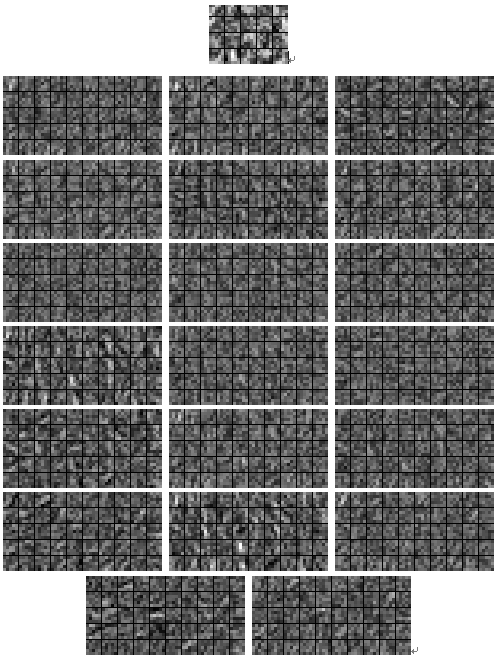## 输入样本并生成特征图

1、首先随意传入一个样本：

res = net.forward({test_x(1,:)});

2、上文中我们都知道，权值数据的获取方式为：

weight = net.layers(layernames{li}).params(1).get_data();

featmap = net.blobs(layernames{li}).get_data();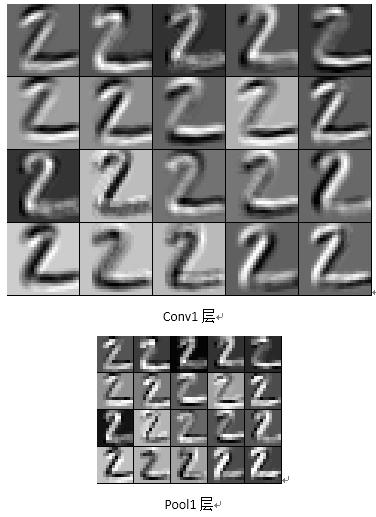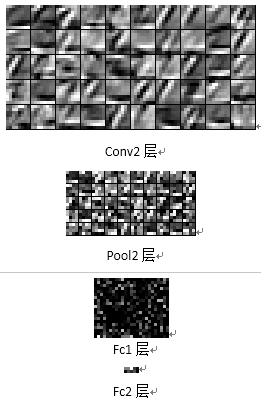### 作者的其它热门文章

0
18 收藏

0 评论
18 收藏
0